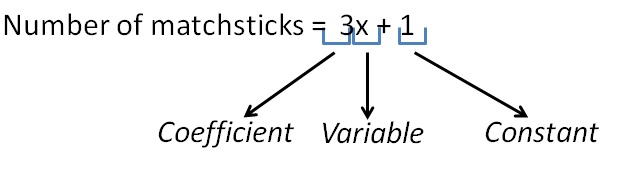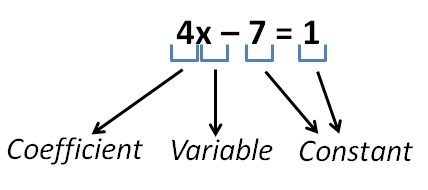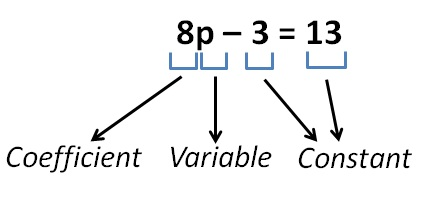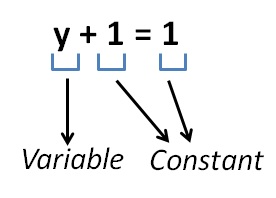Variable

Chapter 11 Class 6 Algebra
Concept wise

Variable is something whose value can change

Suppose we have an equationHere

• x is a variable
• Number multiplied to x is coefficient – 3
• And 1 is a constant

Similarly,

For equationHere, variable is x

For equationHere, variable is p

Similarly,

For equationHere, variable is y

So, we can use any letter as variable

Let’s check some more examples of variables

Learn in your speed, with individual attention - Teachoo Maths 1-on-1 Class